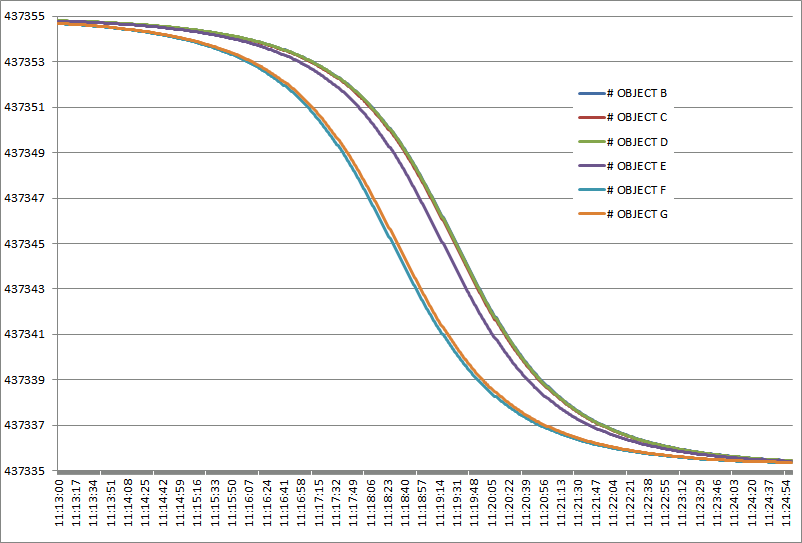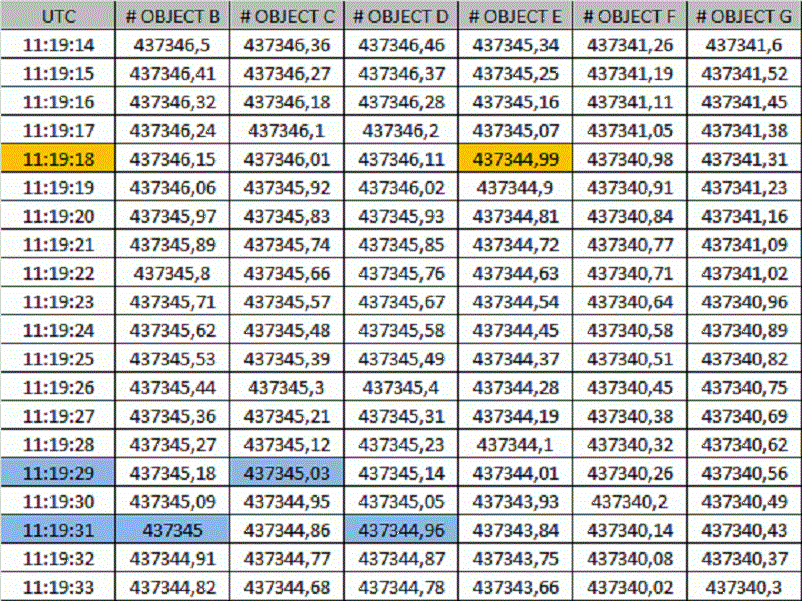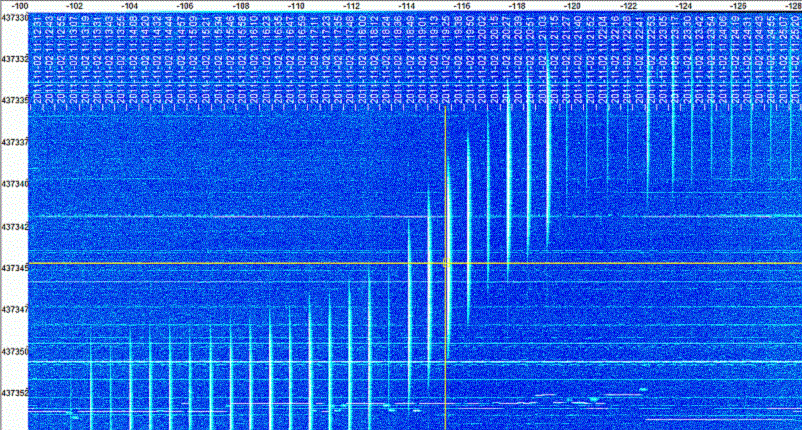# RAX-2 is OBJECT E

A good explanation from DK3WN on how to determine what TLE data belongs to what Satellite.

Based on the latest cubesat TLEs we have now three groups of objects (B+C+D, E, F+G). The first group are the 1U cubesats – they are only a few km apart. In 13 sec distance is RAX-2. I think F and G are the DICE sats.A simple way to determine the TLE, Take one beacon frequency – in this case 437.435 MHz (belongs to RAX-2). Then I calculated all the doppler frequencies for all objectes on a high 66 deg elevation pass. If the doppler = 0 then we have TCA. In this way you can calculate the distance between the TCA of each satellite.You can see the 13 sec difference of RAX to to the first group (E1P, MCubed and AubieSat-1)Take a look at the spectrogram – the horizontal yellow line is the center frequency of 437.345 MHz and the vertical one is the calculated time for RAX-2. And as chance would have it – we have a packet there. If you take the middle frequency of this 9k6 packet -> it all comes to one point.

Source: Satblog – DK3WN